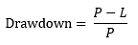To use chat, please login.
Back to contacts

# Drawdown Calculator

 Periods Starting Balance Ending Balance Total Loss Total Loss (%) - - - - -

## How to use the calculator?

Simply enter the starting balance, the number of consecutive losses and the loss per trade (in percent) to calculate the expected drawdown.

## What is Drawdown?

Drawdown is one of the key factors when assessing a trading systems risk. You may often hear the phrase "there is no gain without risk" however how much of a risk is too much?
With a drawdown value you can quickly know how much of a risk the investment has taken.
Drawdown is the highest drop from peak (highest point) to valley (lowest point) in either percentage or money value.
For example, an investment with a 50% drawdown means that it had a realized or non-realized loss of 50% of the investments value at some point.

## What is the formula for Drawdown?P = Peak Balance

L = Lowest Balance (valley)

## How to calculate Drawdown?

Lets say your account hits a high balance of \$100 and drops down to \$72. That is a drawdown of (100-72)/100=28%.

Every time the account hits a new peak, you look for a new low point to calculate the new drawdown. If youre getting a higher drawdown value than the previous value, you have a new max drawdown.

## Why Drawdown is important?

When you compare 2 trading systems, a trading system with a higher return doesnt necessarily mean a better trading strategy - it might simply mean that it took bigger risks than the lower return system. If you wish to know which system has a better risk to reward ratio, you can simply divide the return by drawdown - the higher value will be the trading system that took less risk for the same return.

For example:

• trading system (a) with a 50% gain and 10% drawdown has a risk ratio of 5
• trading system (b) with a 70% gain and a 25% drawdown has a risk ratio of 2.8

So even though system (b) made higher return, it took almost as twice the risk as system (a). Some investors would prefer higher return however some will prefer to keep risk to the minimum and so will prefer system (a).